Function Repository Resource:

# Mediant

Compute the mediant of a list of non-negative rationals

Contributed by: William Gosper (with additional contributions by the Wolfram Function Repository Team)
 ResourceFunction["Mediant"][{r1,r2,…}] gives the sum of the numerators of the ri divided by the sum of their denominators.

## Details

ResourceFunction["Mediant"] will only evaluate if the input is a list of non-negative rational numbers (this includes integers).
The mediant of a list of positive rationals will always be between the smallest and the largest in the list.

## Examples

### Basic Examples (1)

Take the mediant of a pair of fractions:

 In:=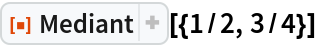Out=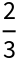### Properties and Relations (2)

Create a list of pseudorandom positive rationals:

 In:=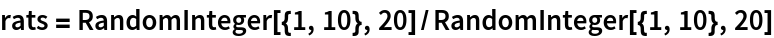Out=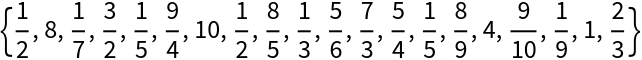Take the mediant:

 In:=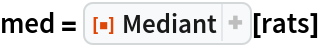Out=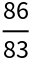Check that the mediant lies between the smallest and largest:

 In:=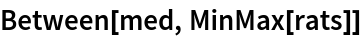Out=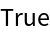In a Farey sequence, the kth element is the mediant of the (k-1)th and (k+1)th elements:

 In:=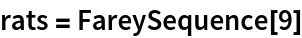Out=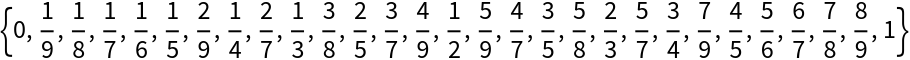In:=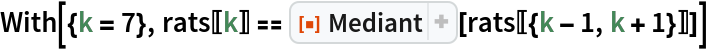Out=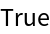The value of the Minkowski question mark function at the mediant can be expressed in terms of the values at the Farey neighbors:

 In:=Out=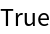### Possible Issues (1)

Mediant will not work with numbers that are not explicitly non-negative integers or rationals:

 In:=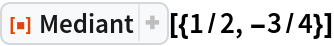Out=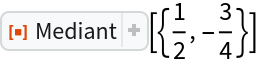## Version History

• 1.1.0 – 24 August 2021
• 1.0.0 – 03 April 2020